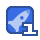• ### lex一个词法分析器的生成器_翻译版

lex一个词法分析器的生成器_翻译版，编译原理课程的上机题目

2013-05-31
38
• ### 语法分析代码

语法分析代码，很详细，可以顺利运行，编译原理的

2013-05-31
3
• ### 计算机组成原理习题集

一．填空题 1.A.10000 B.神威 C.美国，日本 2. A.符号位S B.真值e C.偏移量 3. A.瞬间启动 B.存储器 C.固态盘 4. A.时间 B.空间 C.时间并行+空间 5. A.主设备 B.控制权 C.总线仲裁 6. A.磁光盘 B.相光盘 C.随机写入，擦除或重写 二. 解：设最高位为符号位，输入数据为[x]原 = 01111 [y]原 = 11101 因符号位单独考虑，尾数算前求补器输出值为：|x| = 1111, |y| = 1101 乘积符号位运算： x0 ⊕y0 = 0⊕1 =1 尾数部分运算： 1 1 1 1 × 1 1 0 1 1 1 1 1 0 0 0 0 1 1 1 1 1 1 1 1 1 1 0 0 0 0 1 1 经算后求补器输出，加上乘积符号位，得原码乘积值[x×y] 原 = 111000011 换算成二进制真值 x×y = (-11000011)2 = (-195)10 十进制数乘法验证：x×y = 15×(-13) = -195 三．解：运算器的故障位置在多路开关B,其输出始终为R1的值。分析如下： ⑴ R1(A)+R2(B)=1010，输出结果错； ⑵ R2(A)+R1(B)=1111，结果正确，说明R2(A)，R1(B)无错； ⑶ R1(A)+R1(B)=1010，结果正确，说明R1(A)，R1(B)无错。由此可断定ALU和BR无错； ⑷ R2(A)+R2(B)=1111。结果错。由于R2(A)正确，且R2(A)=1010，本应R2(B)=1010，但此时推知R2(B)=0101，显然，多路开关B有问题； ⑸ R2(A)+BR(B)=1111，结果错。由于R2(A)=1010，BR(B)=1111，但现在推知BR(B)=0101，证明开关B输出有错； ⑹ R1(A)+BR(B)=1010，结果错。由于R1(A)=0101,本应BR(B)=1111，但现在推知BR(B)=0101，仍证明开关B出错。 综上所述，多路开关B输出有错。故障性质：多路开关B输出始终为0101。这有两种可能：一是控制信号BS0,BS1始终为01，故始终选中寄存器R1；二是多路开关B电平输出始终嵌在0101上。

2012-10-13
3
• 3
资源
• 0
粉丝
•等级